Question

# The enthalpy of fusion of bismuth at its normal melting point of 271 °C is 11.0...

The enthalpy of fusion of bismuth at its normal melting point of 271 °C is 11.0 kJ mol-1. What is the entropy of fusion of bismuth at this temperature?

ΔSfus =  J mol-1 K-1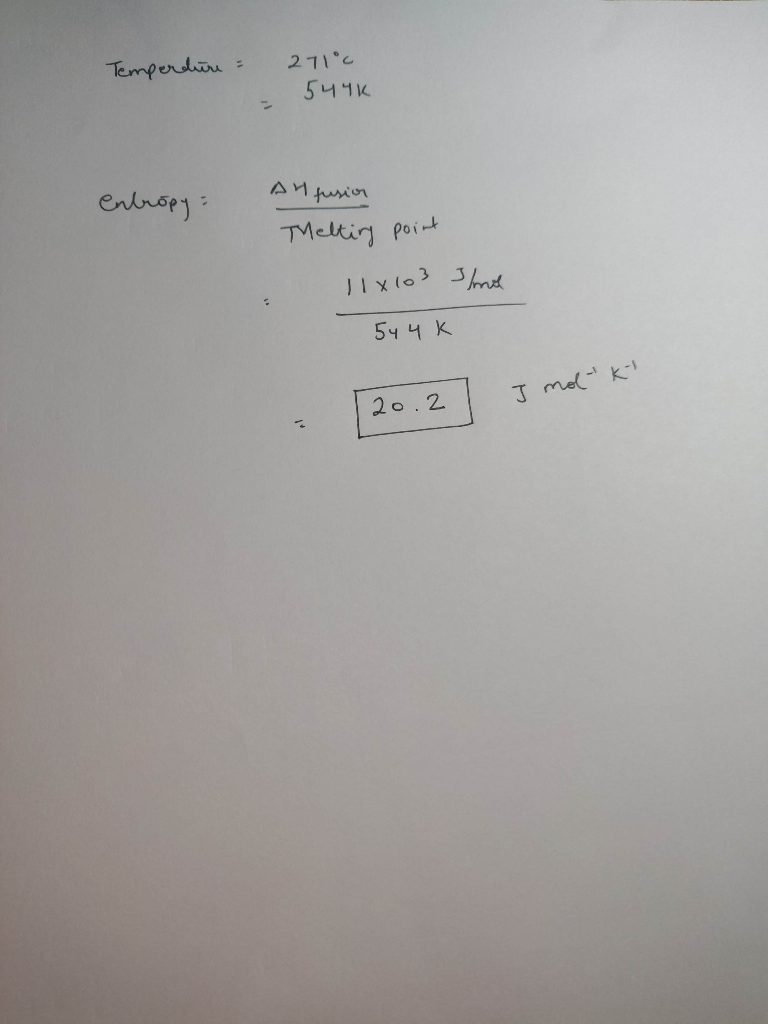#### Earn Coins

Coins can be redeemed for fabulous gifts.

Similar Homework Help Questions
• ### 14. For bismuth, Bi, the heat of fusion at its normal melting point of 271 °C...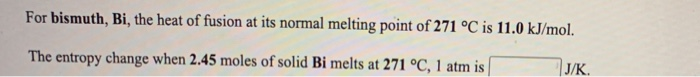14. For bismuth, Bi, the heat of fusion at its normal melting point of 271 °C is 11.0 kJ/mol. The entropy change when 2.45 moles of solid Bi melts at 271 °C, 1 atm is J/K. For magnesium, Mg, the heat of fusion at its normal melting point of 649 °C is 9.0 kJ/mol. The entropy change when 1.72 moles of liquid Mg freezes at 649 °C, 1 atm is J/K

• ### The molar enthalpy of fusion of solid bismuth is 11.0 kJ mol-1, and the molar entropy...

The molar enthalpy of fusion of solid bismuth is 11.0 kJ mol-1, and the molar entropy of fusion is 20.2 J K-1 mol-1. (a) Calculate the Gibbs free energy change for the melting of 1.00 mol of bismuth at 575 K. kJ (b) Calculate the Gibbs free energy change for the conversion of 5.12 mol of solid bismuth to liquid bismuth at 575 K. kJ (c) Will bismuth melt spontaneously at 575 K? (d) At what temperature are solid and...

• ### The enthalpy of fusion of cadmium at its normal melting point of 321 °C is 6.11...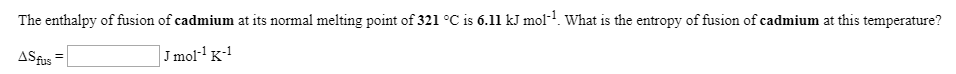The enthalpy of fusion of cadmium at its normal melting point of 321 °C is 6.11 kJ mol? What is the entropy of fusion of cadmium at this temperature? ASfus = J mol-K-1 The molar enthalpy of fusion of solid cadmium is 6.11 kJ mol-1, and the molar entropy of fusion is 10.3 JK+mol-1. (a) Calculate the Gibbs free energy change for the melting of 1.00 mol of cadmium at 622 K. (b) Calculate the Gibbs free energy change for...

• ### The enthalpy of fusion of manganese at its normal melting point of 1244 ? is 14.6...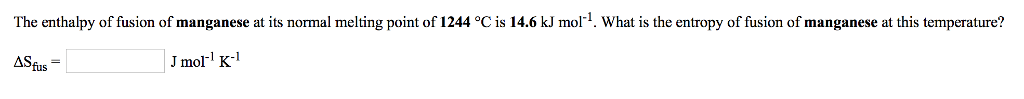The enthalpy of fusion of manganese at its normal melting point of 1244 ? is 14.6 kJ mol-1 What is the entropy of fusion of manganese at this temperature? ASfus Jmol K

• ### For ethanol, C2H5OH, the heat of fusion at its normal melting point of -115 °C is...

For ethanol, C2H5OH, the heat of fusion at its normal melting point of -115 °C is 5.0 kJ/mol. The entropy change when 1.84 moles of solid C2H5OH melts at -115 °C, 1 atm is J/K.

• ### For copper, Cu, the heat of fusion at its normal melting point of 1083 °C is...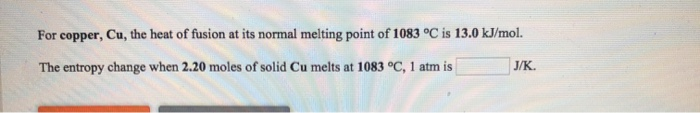For copper, Cu, the heat of fusion at its normal melting point of 1083 °C is 13.0 kJ/mol. The entropy change when 2.20 moles of solid Cu melts at 1083 °C, 1 atm is J/K

• ### The enthalpy of fusion for benzene (C6H6) is 9.937 kJ/mol, and the entropy change for fusion...

The enthalpy of fusion for benzene (C6H6) is 9.937 kJ/mol, and the entropy change for fusion is 35.7 J/(mol K). What is the normal melting point for benzene in degree Celsius?

• ### The normal melting point of sodium chloride is 801°C, its enthalpy of fusion is 28.8 kJ/mol,...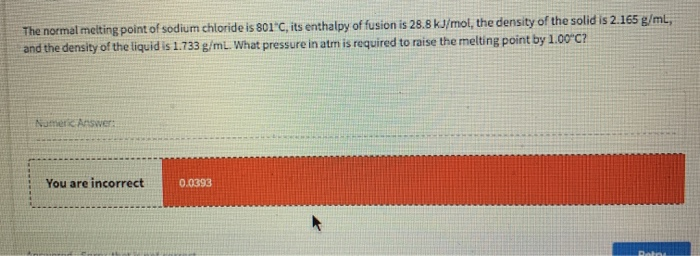The normal melting point of sodium chloride is 801°C, its enthalpy of fusion is 28.8 kJ/mol, the density of the solid is 2.165 g/ml, and the density of the liquid is 1.733 g/ml. What pressure in atm is required to raise the melting point by 1.00°C? Nume Answer: You are incorrect 0.0393

• ### For bismuth, Bi, the heat of vaporization at its normal boiling point of 1627 °C is...

For bismuth, Bi, the heat of vaporization at its normal boiling point of 1627 °C is 172.0 kJ/mol. The entropy change when 2.11 moles of Bi vapor condenses at 1627 °C, 1 atm is J/K.

• ### 6. The melting point of CaTiSiOs is 1400°C and the heat of fusion at the normal melting point is 123 700 J mol-1. Calculate the heat of fusion at 1300°C. Cnsolid = 177.4 + 23.2 x 10', 40.3 x...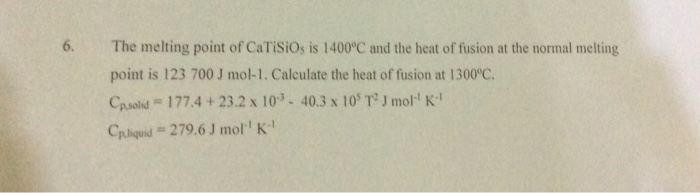6. The melting point of CaTiSiOs is 1400°C and the heat of fusion at the normal melting point is 123 700 J mol-1. Calculate the heat of fusion at 1300°C. Cnsolid = 177.4 + 23.2 x 10', 40.3 x 105 T2 J mor' K" Cphquid # 279.6 J mol'' K" 6. The melting point of CaTiSiOs is 1400°C and the heat of fusion at the normal melting point is 123 700 J mol-1. Calculate the heat of fusion at 1300°C....